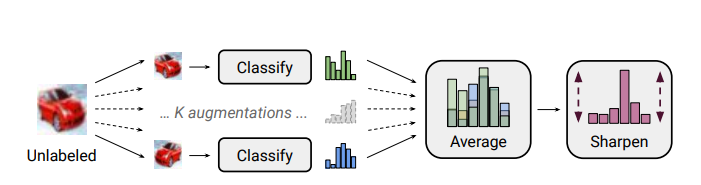# Two new Semi-supervised learning methods from Google MixMatch and UDA

Semi-supervised learning (SSL) seeks to largely alleviate the need for labeled data by allowing a model to leverage unlabeled data. Many recent approaches for semi-supervised learning add a loss term which is computed on unlabeled data and encourages the model to generalize better to unseen data.

In much recent work, this loss term falls into one of three classes:

• Consistency regularization applies data augmentation to semi-supervised learning by leveraging the idea that a classifier should output the same class distribution for an unlabeled example even after it has been augmented.
• A common underlying assumption in many semi-supervised learning methods is that the classifier’s decision boundary should not pass through high-density regions of the marginal data distribution. One way to enforce this is to require that the classifier output low-entropy predictions on unlabeled data.
• Regularization refers to the general approach of imposing a constraint on a model to make it harder to memorize the training data and therefore hopefully make it generalize better to unseen data

# MixMatch: A Holistic Approach to Semi-Supervised LearningMixMatch utilizes MixUp regularizer, which trains a model on convex combina- tions of both inputs and labels. MixUp can be seen as encouraging the model to have strictly linear behavior “between” examples, by requiring that the model’s output for a convex combination of two inputs is close to the convex combination of the output for each individual input.

MixMatch contains the following steps:

• augment the unlabled data (label is unchanged) for k rounds
• guessing for each round of augmented data
• averaging and sharpening (similar to softmax) the label for the unlabled data
• augment the labeled data (label is unchanged)
• use MixUp to combine the augmented labeled data and augmented unlabeled data
• use the combined data to train the data.

The Mixup is very simple: $$\lambda = \Beta(\alpha, \alpha)\\ \hat(\lambda) = \max{(\lambda, 1-\lambda)} \hat(x_i) = \hat(\lambda)x_i^L + (1-\hat(\lambda))x_i^U \hat(y_i) = \hat(\lambda)y_i^L + (1-\hat(\lambda))y_i^U$$

According to the experiment, mix up (especially with unlabled data) and sharpening are critical to the performance.

# Unsupervised Data AugmentationUnsupervised Data Augmentation (UDA) encourages the model predictions to be consistent (measured via KL divergence) between an unlabeled example and an augmented unlabeled example. However, instead of using conventional perturbations such as Gaussian noise, dropout noise or affine transformations, UDA proposed to learn a task-specific perturbations.

The augmentation method could be:

• AutoAugment for image classification
• Cutout for image classification
• Back translation for text classification, e.g., english to french then french to english
• TF-IDF for text classification: replacing the words which are not keywords

Training Signal Annealing (TSA) is then proposed to handle the overfitting. The main intuition behind TSA is to gradually release the training signals of the supervised examples as the model is trained on more and more unsupervised examples.

Written on June 7, 2019